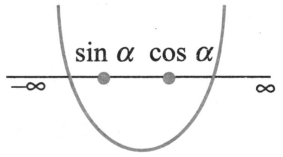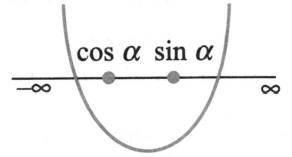Theory of expressions
Question

# Consider equation $\left(\mathrm{x}-\mathrm{sin}\mathrm{\alpha }\right)\left(\mathrm{x}-\mathrm{cos}\mathrm{\alpha }\right)-2=0$. Which of the following is/are true?

Moderate
Solution

## Let $\mathrm{f}\left(\mathrm{x}\right)=\left(\mathrm{x}-\mathrm{sin}\mathrm{\alpha }\right)\left(\mathrm{x}-\mathrm{cos}\mathrm{\alpha }\right)-2$.Then, $\mathrm{f}\left(\mathrm{sin}\mathrm{\alpha }\right)=-2<0$ and $\mathrm{f}\left(\mathrm{cos}\mathrm{\alpha }\right)=-2<0$.So,  lie between the roots.For $0<\mathrm{\alpha }<\frac{\mathrm{\pi }}{4},\mathrm{sin}\mathrm{\alpha }<\mathrm{cos}\mathrm{\alpha }$Therefore, equation f (x) = 0 has one root in  and other in .Also, for $\frac{\mathrm{\pi }}{4}<\mathrm{\alpha }<\frac{\mathrm{\pi }}{2}$, $\mathrm{cos}\mathrm{\alpha }<\mathrm{sin}\mathrm{\alpha }$Therefore, equation f(x) = 0 has one root in  and other in .

Get Instant Solutions